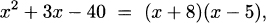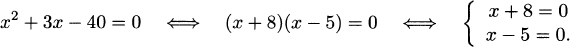# MATRIX FACTORIZATIONS

We are very familiar with factorizations of one-dimensional algebraic expressions such asand in a very natural way we use such factorizations to solve algebraic equations like x2 + 3x − 40 = 0. The idea behind this, is to transform the problem of solving one quadratic equation into the problem of solving two (much simpler) linear equations:It is very pleasant (and at this point maybe not surprising) to know that this idea generalizes to higher dimensions. More specifically, when we are faced with the problem of solving equations involving arbitrary matrices, that problem can be transformed into a simpler one via certain matrix factorization. For instance, as we will see in detail later on, we can use the LU factorization of a matrix A to transform the problem of solving a system of equations Ax = b into solving two (much simpler) upper and lower triangular systems:But there is more than that. Factorization of matrices is also useful because it uncovers several properties of the matrices and of special sets of vectors associated with them, which are “invisible” without such factorization, and this has a great number of applications not only within ...

Get A First Course in Applied Mathematics now with the O’Reilly learning platform.

O’Reilly members experience live online training, plus books, videos, and digital content from nearly 200 publishers.